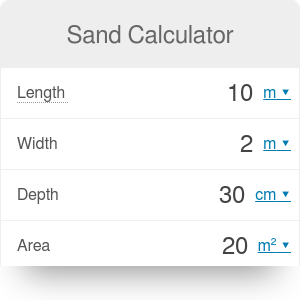Length
ft
Width
ft
Depth
in
Area
ft²
Volume needed
cu yd
Density
lb/cu ft
Weight needed
US ton
Price
\$/
US ton
Price
\$/
cu yd
Total cost
\$

# Sand Calculator

By Bogna Haponiuk

Our sand calculator is a tool designed specifically to help you with calculations you may do on the building site, or when you are doing a bit of home improvement. Estimating the required amount of any building material is a difficult task, and errors may result in either the material running out when the project is in full swing, or in heaps of sand lying around after the earthwork is completed. Use this sand calculator or our paver sand calculator to answer the question "how much sand do I need" and never worry about it again!

## How much sand do I need?

The first step is to establish the volume of sand needed. It is equal to the volume of excavation, and you can do it with the following method:

1. Determine the length and width of the cuboidal excavation. For example, we can assume an excavation of length `L = 12 yd` and width `b = 3 yd`.
2. Calculate the area of the excavation by multiplying the length by the width. In our case, `A = 12 * 3 = 36 yd2`. You can also type the area of the excavation directly into our calculator if you choose an excavation of some more sophisticated shape.
3. Establish the depth of the excavation. Let's say it's `d = 0.5 yd`.
4. Multiply the area by the depth of the excavation to obtain its volume: `36 * 0.5 = 18 cu yd`.
5. The volume of sand required is equal to the volume of excavation. Our sand calculator will display this value for you.

## All right, but how much does a yard of sand weigh?

To calculate the weight of a cubic yard of sand, you simply have to multiply its volume by its density. You don't have to remember the density of sand though - our calculator has a preset value for density. Of course, if you decide to use some unusual material, feel free to change the value!

Once you know the total weigh of sand you have to buy, you won't have to worry about overspending on building materials. But exactly how much will you spend? Our sand calculator can help you with that - all you have to do is enter the price of sand (per unit of mass, such as tonne, or per unit of volume, such as cubic yard). The calculator will then display the total cost of the sand you need.

## What if I use concrete?

Then head to our concrete estimator to calculate the number of bags of cement needed to cast the concrete element of your design.

Bogna Haponiuk

## Get the widget!

Sand Calculator can be embedded on your website to enrich the content you wrote and make it easier for your visitors to understand your message.

It is free, awesome and will keep people coming back!